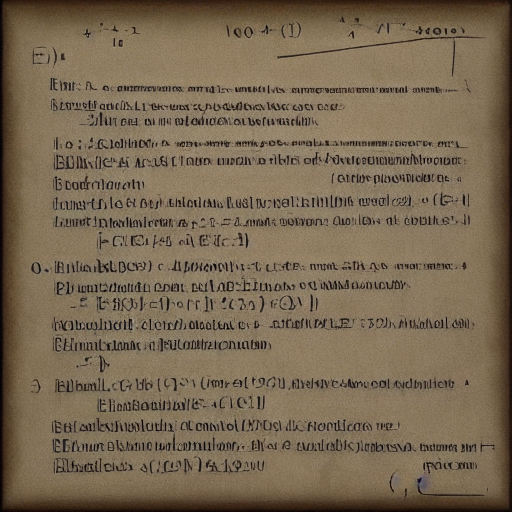### Eulers Theorem

Groups make it easy

Update: This is all a trivial consequence of Lagrange's Theorem on finite groups implying groups of prime order are all the cyclic group. See this video for a great explanation.

Eulers theorem: If $\phi(n)$ is the number of integers coprime to $n$, and $k$ is any integer coprime to $n$ we have

$k^{\phi(n)} \equiv 1 \pmod n$

## Key fact

The integers coprime to n, mod n forms a group (generally denoted $(\mathbb{Z}/n\mathbb{Z})^{\times}$). Since multiplication by $k$ is invertible (because it's a group) multiplying each element by $k$ just reorders the elements.

Example: multiply each coprime number by $3$ mod $8$ (Remember mod is the same as the remainder from divison)

\begin{aligned} (1 \cdot 3) &= 3 &\to 3 \\ (3 \cdot 3) &= 9 &\to 1 \\ (5 \cdot 3) &= 15 &\to 7 \\ (7 \cdot 3) &= 21 &\to 5 \end{aligned}

And the product is the same if each element is multiplied by $3$, since we take out as many $8$'s as we can from each term.

$1 \cdot 3 \cdot 5 \cdot 7 \equiv (3 \cdot 1) (3 \cdot 3) (3 \cdot 5) (3 \cdot 7) \equiv 3 \cdot 1 \cdot 7 \cdot 5 \pmod 8$

## The Proof

Let $\{k_1, \dots, k_{\phi(n)}\}$ be the integers coprime to $n$ and let $k$ be coprime to $n$.

We know $k$ is in the set (since n and k are coprime). if we multiply each $k_i$ by $k$ we have

$\prod^{\phi(n)} k_i \equiv \prod^{\phi(n)} k_i k \pmod n$

Because of invertibility (no overlaps/no duplicates) and closure (stays in the set of coprimes), multiplying each $k_i$ by $k$ just reorders the elements.

Now we factor out k and invert/divide by the product (which will just be another k)

$1 \cdot \bcancel{\prod^{\phi(n)} k_i} \equiv k^{\phi(n)} \bcancel{\prod^{\phi(n)} k_i} \pmod n$

So we end up with

$k^{\phi(n)} \equiv 1 \pmod n$

## Proof it is a group

There are 4 group axioms we must satisfy

1. Closure: if $k_1$ and $k_2$ share no factors with $n$, $k_1 k_2$ share no factors with $n$.
2. An identity element: just $1$ in our case
3. Associativity: multiplication is associative
4. Invertibility: see below

Let $k$ be an element of the group, we know that $\gcd(k, n) = 1$ (coprime)

Euclids algorithm implies there exist $s$ and $t$ such that

$sk + tn = 1$

(Also known as Bézout's identity)

Now mod out by $n$

$sk + tn = 1 \implies sk \equiv 1 \pmod n$

Therefor $s = k^{-1}$, and an inverse exists!

## General case (Group Theory)

Looking back on this proof, the only facts we needed were that $(\mathbb{Z}/n\mathbb{Z})^{\times}$ is a finite group, and that multiplication is communicative (needed when we factored out $k^{\phi(n)}$). Since those are the only dependencies, it must be true for all finite communicative groups!

An example is the additive group of integers modulo $n$ denoted $(\mathbb{Z}/n\mathbb{Z})^+$, in this case Group multiplication becomes Group addition, the identity element becomes $0$ and $x^n$ now denotes repeated addition so $x^n = nx$.

Our theorem says that $x^n = 1$ for all $x$ in the group, where $n$ is the size of the group. In the addition case this becomes $nx = 0 \pmod n$ which can be seen directly as $nx$ is divisible by $n$.